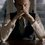# Calculus Challenge 5!

Define ${f}_{a}^{b}(x)$ as a function which converts $x$ into base $a$ and then interprets it as a number in base $b$.

For example,

${f}_{2}^{10}(0.5)$ will mean first changing $0.5$ to base $2$ i.e. $0.1$ and then interpreting $0.1$ as a base $10$ number. That's it!

So, find a general formula for

$\displaystyle \int_{0}^{1}{{f}_{b}^{a}(x) \ dx}$

I will not be giving the form for this problem as then the difficulty will be halved so try yourself and then if required I will post the solution as the contest ends.Note by Kartik Sharma
5 years, 11 months ago

This discussion board is a place to discuss our Daily Challenges and the math and science related to those challenges. Explanations are more than just a solution — they should explain the steps and thinking strategies that you used to obtain the solution. Comments should further the discussion of math and science.

When posting on Brilliant:

• Use the emojis to react to an explanation, whether you're congratulating a job well done , or just really confused .
• Ask specific questions about the challenge or the steps in somebody's explanation. Well-posed questions can add a lot to the discussion, but posting "I don't understand!" doesn't help anyone.
• Try to contribute something new to the discussion, whether it is an extension, generalization or other idea related to the challenge.

MarkdownAppears as
*italics* or _italics_ italics
**bold** or __bold__ bold
- bulleted- list
• bulleted
• list
1. numbered2. list
1. numbered
2. list
Note: you must add a full line of space before and after lists for them to show up correctly
paragraph 1paragraph 2

paragraph 1

paragraph 2

[example link](https://brilliant.org)example link
> This is a quote
This is a quote
    # I indented these lines
# 4 spaces, and now they show
# up as a code block.

print "hello world"
# I indented these lines
# 4 spaces, and now they show
# up as a code block.

print "hello world"
MathAppears as
Remember to wrap math in $$ ... $$ or $ ... $ to ensure proper formatting.
2 \times 3 $2 \times 3$
2^{34} $2^{34}$
a_{i-1} $a_{i-1}$
\frac{2}{3} $\frac{2}{3}$
\sqrt{2} $\sqrt{2}$
\sum_{i=1}^3 $\sum_{i=1}^3$
\sin \theta $\sin \theta$
\boxed{123} $\boxed{123}$

Sort by:

So, now I guess I must post the solution.

First of all what does $f_b^a (x)$ means? For $x<1$, $\displaystyle {f}_{b}(x) = \sum_{k=1}^{\infty}{\left \lfloor b^k x \right \rfloor \pmod{b}}$ and

$\displaystyle f_b^a(x) = \sum_{k=1}^{\infty}{\frac{\left \lfloor b^k x \right \rfloor \pmod{2}}{a^k}}$

$\displaystyle \int_{0}^{1}{\sum_{k=0}^{\infty}{\frac{\left \lfloor b^k x \right \rfloor \pmod{b}}{a^k}} \ dx}$

$\displaystyle \sum_{k=1}^{\infty}{\frac{1}{a^k} \int_{0}^{1}{\left \lfloor b^k x \right \rfloor \pmod{b} \ dx}}$

$\displaystyle \text{Lemma} : \int_{0}^{1}{\left \lfloor b^k x \right \rfloor \pmod{b} \ dx} = \frac{b-1}{2}$

$\displaystyle \text{Proof} :$ First substitute $u = b^k x$ and then draw a graph for $\displaystyle u \pmod{b}$ from $u = 0$ to $u = b^k$. You will find that it is periodic with period $b$ and in each period, there are $1 \times k$ rectangles where $\displaystyle k = [1,2,\cdots, b-1]$.

Hence, the area under the graph will just be the area of these rectangles i.e. in one period the area is $\displaystyle 1 \times 1 + 1 \times 2 + 1 \times 3 + \cdots + 1 \times (b-1) = \frac{b(b-1)}{2}$ and there are $\displaystyle {b}^{k-1}$ "oscillations". As a result our total area becomes $\displaystyle {b}^{k-1} \frac{b(b-1)}{2} = \frac{b^k(b-1)}{2}$. So, our integral which is just $\displaystyle \frac{1}{b^k}$ of this total area becomes $\displaystyle \frac{b-1}{2}$.

Now, we get

$\displaystyle \sum_{k=1}^{\infty}{\frac{1}{a^k} \frac{b-1}{2}}$

$\large \displaystyle = \boxed{\frac{b-1}{2(a-1)}}$

This is original.

- 5 years, 10 months ago

There can be more generalizations to this as well. For example, considering $a,b$ to have non-integer values or changing the limits of the problem and so on. Take these up as challenges now!

- 5 years, 10 months ago

Can u provide the solution?? I couldn't get any.

- 5 years, 11 months ago

It's one of my favorites. I will surely post solutions for all the unanswered challenges as soon as the contest ends.

- 5 years, 11 months ago

Ok. I'll give it a try again.

- 5 years, 11 months ago

Do we assume $a$ and $b$ to be positive integers?

- 5 years, 11 months ago

Oh yeah I forgot to mention that. I apologize for that.

- 5 years, 11 months ago

In $f_a^b(x)$, is $a\le b$? If no, how can we interpret $3$ in base $2$?

- 5 years, 11 months ago

Okay, you may assume that.

- 5 years, 11 months ago